# Check if a string is a subsequence of another string ( using Stacks )

Given a string S, the task is to check if the string target is a subsequence of string S or not, using a Stack.

Examples:

Input: S = ”KOTTAYAM”, target = ”KOTA”
Output: Yes
Explanation: “KOTA” is a subsequence of “KOTTAYAM”.

Input: S = ”GEEKSFORGEEKS”, target =”FORFOR”
Output: No

Approach: Follow the steps to solve the problem: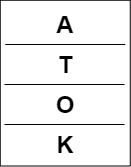target pushed into the stack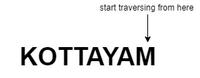Traversing in S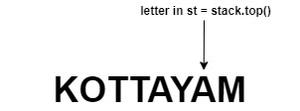Traversing in S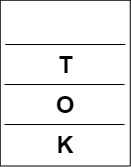Popping from stack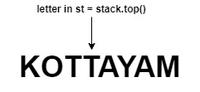Traversing in S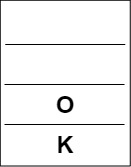Popping from stack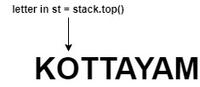Traversing in st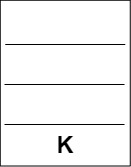Popping from stack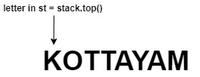Traversing in S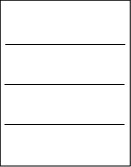Stack becomes empty

Below is the implementation of the above approach:

## C++

 `// C++ Program for the above approach`   `#include ` `using` `namespace` `std;`   `// Function to check if target` `// is a subsequence of string S` `void` `checkforSubsequence(string S,` `                         ``string target)` `{`   `    ``// Declare a stack` `    ``stack<``char``> s;`   `    ``// Push the characters of` `    ``// target into the stack` `    ``for` `(``int` `i = 0; i < target.size(); i++) {` `        ``s.push(target[i]);` `    ``}`   `    ``// Traverse the string S in reverse` `    ``for` `(``int` `i = (``int``)S.size() - 1; i >= 0; i--) {`   `        ``// If the stack is empty` `        ``if` `(s.empty()) {`   `            ``cout << ``"Yes"` `<< endl;` `            ``return``;` `        ``}`   `        ``// if S[i] is same as the` `        ``// top of the stack` `        ``if` `(S[i] == s.top()) {`   `            ``// Pop the top of stack` `            ``s.pop();` `        ``}` `    ``}`   `    ``// Stack s is empty` `    ``if` `(s.empty())` `        ``cout << ``"Yes"` `<< endl;` `    ``else` `        ``cout << ``"No"` `<< endl;` `}`   `// Driver Code` `int` `main()` `{` `    ``string S = ``"KOTTAYAM"``;` `    ``string target = ``"KOTA"``;`   `    ``checkforSubsequence(S, target);`   `    ``return` `0;` `}`

## Java

 `// Java approach for the above approach` `import` `java.util.Stack;`   `public` `class` `GFG {`   `    ``// Function to check if target` `    ``// is a subsequence of string S` `    ``static` `void` `checkforSubsequence(String S, String target)` `    ``{`   `        ``// Declare a stack` `        ``Stack s = ``new` `Stack<>();`   `        ``// Push the characters of` `        ``// target into the stack` `        ``for` `(``int` `i = ``0``; i < target.length(); i++) {` `            ``s.push(target.charAt(i));` `        ``}`   `        ``// Traverse the string S in reverse` `        ``for` `(``int` `i = (``int``)S.length() - ``1``; i >= ``0``; i--) {`   `            ``// If the stack is empty` `            ``if` `(s.empty()) {`   `                ``System.out.println(``"Yes"``);` `                ``return``;` `            ``}`   `            ``// if S[i] is same as the` `            ``// top of the stack` `            ``if` `(S.charAt(i) == s.peek()) {`   `                ``// Pop the top of stack` `                ``s.pop();` `            ``}` `        ``}`   `        ``// Stack s is empty` `        ``if` `(s.empty())` `            ``System.out.println(``"Yes"``);` `        ``else` `            ``System.out.println(``"No"``);` `    ``}`   `    ``// Driver Code` `    ``public` `static` `void` `main(String[] args)` `    ``{` `        ``String S = ``"KOTTAYAM"``;` `        ``String target = ``"KOTA"``;`   `        ``checkforSubsequence(S, target);` `    ``}` `}`   `// This code is contributed by abhinavjain194`

## Python3

 `# Python3 program for the above approach`   `# Function to check if target` `# is a subsequence of string S` `def` `checkforSubsequence(S, target):`   `    ``# Declare a stack` `    ``s ``=` `[]`   `    ``# Push the characters of` `    ``# target into the stack` `    ``for` `i ``in` `range``(``len``(target)):` `        ``s.append(target[i])`   `    ``# Traverse the string S in reverse` `    ``for` `i ``in` `range``(``len``(S) ``-` `1``, ``-``1``, ``-``1``):`   `        ``# If the stack is empty` `        ``if` `(``len``(s) ``=``=` `0``):` `            ``print``(``"Yes"``)` `            ``return`   `        ``# If S[i] is same as the` `        ``# top of the stack` `        ``if` `(S[i] ``=``=` `s[``-``1``]):`   `            ``# Pop the top of stack` `            ``s.pop()`   `    ``# Stack s is empty` `    ``if` `(``len``(s) ``=``=` `0``):` `        ``print``(``"Yes"``)` `    ``else``:` `        ``print``(``"No"``)`   `# Driver Code` `if` `__name__ ``=``=` `"__main__"``:`   `    ``S ``=` `"KOTTAYAM"` `    ``target ``=` `"KOTA"`   `    ``checkforSubsequence(S, target)` `    `  `# This code is contributed by ukasp`

## C#

 `// C# approach for the above approach` `using` `System;` `using` `System.Collections.Generic;`   `class` `GFG{`   `// Function to check if target` `// is a subsequence of string S` `static` `void` `checkforSubsequence(String S, ` `                                ``String target)` `{` `    `  `    ``// Declare a stack` `    ``Stack<``char``> s = ``new` `Stack<``char``>();`   `    ``// Push the characters of` `    ``// target into the stack` `    ``for``(``int` `i = 0; i < target.Length; i++)` `    ``{` `        ``s.Push(target[i]);` `    ``}`   `    ``// Traverse the string S in reverse` `    ``for``(``int` `i = (``int``)S.Length - 1; i >= 0; i--) ` `    ``{`   `        ``// If the stack is empty` `        ``if` `(s.Count == 0) ` `        ``{` `            ``Console.WriteLine(``"Yes"``);` `            ``return``;` `        ``}`   `        ``// If S[i] is same as the` `        ``// top of the stack` `        ``if` `(S[i] == s.Peek())` `        ``{` `            `  `            ``// Pop the top of stack` `            ``s.Pop();` `        ``}` `    ``}`   `    ``// Stack s is empty` `    ``if` `(s.Count == 0)` `        ``Console.WriteLine(``"Yes"``);` `    ``else` `        ``Console.WriteLine(``"No"``);` `}`   `// Driver Code` `public` `static` `void` `Main(String[] args)` `{` `    ``String S = ``"KOTTAYAM"``;` `    ``String target = ``"KOTA"``;`   `    ``checkforSubsequence(S, target);` `}` `}`   `// This code is contributed by shikhasingrajput`

## Javascript

 ``

Output

```Yes

```

Time Complexity : O(N)
Auxiliary Space : O(N)

Approach 2: Using the find() function

Another approach to check if a string is a subsequence of another string is to use the find() function. We can call find() on the second string to find the index of the first occurrence of the first character of the first string. Then, we can iterate over the first string and call find() on the second string with the starting index set to the index of the previous character plus one. If find() returns string::npos, it means that the current character in the first string is not present in the second string and hence the first string is not a subsequence of the second string.

Define a function that takes two string arguments s1 and s2.

Initialize an integer variable index to -1. This will be used to keep track of the starting index for each find() call.

Iterate over each character c in s1 using a range-based for loop.

Call the find() function on s2 with the arguments c and index + 1. The second argument specifies the starting index for the search, which is the index of the previous character plus one.

If find() returns string::npos, which indicates that the character was not found in s2, return false immediately, as s1 is not a subsequence of s2.

If find() returns a valid index, update index to the returned value.

After iterating over all the characters in s1, return true, as s1 is a subsequence of s2.

## C++

 `#include ` `#include `   `using` `namespace` `std;`   `bool` `isSubsequence(string s1, string s2) {` `    ``int` `index = -1;` `    ``for` `(``char` `c : s1) {` `        ``index = s2.find(c, index + 1);` `        ``if` `(index == string::npos) {` `            ``return` `false``;` `        ``}` `    ``}` `    ``return` `true``;` `}`   `int` `main() {` `    ``string s1 = ``"KOTA"``;` `    ``string s2 = ``"KOTTAYAM"``;` `    ``bool` `check =  isSubsequence(s1, s2) ;` `    ``if``(check){` `        ``cout<<``" yes"``;` `    ``}` `    ``else``{` `        ``cout<<``"no"``;` `    ``}` `    ``return` `0;` `}`

## Java

 `import` `java.util.*;`   `public` `class` `GFG {` `  `  `    ``public` `static` `boolean` `isSubsequence(String s1, String s2) {` `      ``// Initialize index to -1 ` `      ``int` `index = -``1``; ` `      `  `      ``// Loop through each character in s1` `        ``for` `(``char` `c : s1.toCharArray()) { ` `          `  `          ``// Find the index of the current character in s2` `            ``index = s2.indexOf(c, index + ``1``); ` `          ``// If the character is not found, return false` `            ``if` `(index == -``1``) { ` `                ``return` `false``;` `            ``}` `        ``}` `        ``return` `true``; ``// All characters are found, return true` `    ``}`   ` ``// Driver Code` `    ``public` `static` `void` `main(String[] args) {` `     ``// input taken` `        ``String s1 = ``"KOTA"``;` `        ``String s2 = ``"KOTTAYAM"``;` `        ``boolean` `check = isSubsequence(s1, s2);` `      ``// Print "yes" if s1 is a subsequence of s2` `        ``if` `(check) {` `            ``System.out.println(``"yes"``); ` `        ``} ` `      ``// Print "no" if s1 is not a subsequence of s2` `      ``else` `{` `            ``System.out.println(``"no"``); ` `        ``}` `    ``}` `}`

## Python3

 `def` `isSubsequence(s1, s2):` `    ``index ``=` `-``1` `    ``for` `c ``in` `s1:` `        ``index ``=` `s2.find(c, index ``+` `1``)` `        ``if` `index ``=``=` `-``1``:` `            ``return` `False` `    ``return` `True`   `s1 ``=` `"KOTA"` `s2 ``=` `"KOTTAYAM"` `check ``=` `isSubsequence(s1, s2)` `if` `check:` `    ``print``(``"yes"``)` `else``:` `    ``print``(``"no"``)`

## C#

 `using` `System;`   `public` `class` `Program` `{` `    ``// Function to check if s1 is a subsequence of s2` `    ``static` `bool` `IsSubsequence(``string` `s1, ``string` `s2)` `    ``{` `        ``int` `index = -1;` `        `  `        ``foreach` `(``char` `c ``in` `s1)` `        ``{` `            ``// Search for the character 'c' in s2, starting from index + 1` `            ``index = s2.IndexOf(c, index + 1);`   `            ``// If 'c' is not found in s2, return false` `            ``if` `(index == -1)` `            ``{` `                ``return` `false``;` `            ``}` `        ``}` `        `  `        ``return` `true``;` `    ``}`   `    ``public` `static` `void` `Main()` `    ``{` `        ``string` `s1 = ``"KOTA"``;` `        ``string` `s2 = ``"KOTTAYAM"``;`   `        ``// Check if s1 is a subsequence of s2` `        ``bool` `check = IsSubsequence(s1, s2);`   `        ``// Print the result` `        ``if` `(check)` `        ``{` `            ``Console.WriteLine(``"Yes, s1 is a subsequence of s2"``);` `        ``}` `        ``else` `        ``{` `            ``Console.WriteLine(``"No, s1 is not a subsequence of s2"``);` `        ``}` `    ``}` `}`

## Javascript

 `function` `isSubsequence(s1, s2) {` `    ``// Initialize index to -1` `    ``let index = -1;`   `    ``// Loop through each character in s1` `    ``for` `(const c of s1) {` `        ``// Find the index of the current character in s2` `        ``index = s2.indexOf(c, index + 1);`   `        ``// If the character is not found, return false` `        ``if` `(index === -1) {` `            ``return` `false``;` `        ``}` `    ``}` `    ``return` `true``; ``// All characters are found, return true` `}`   `// Driver Code` `const s1 = ``"KOTA"``;` `const s2 = ``"KOTTAYAM"``;` `const check = isSubsequence(s1, s2);`   `// Print "yes" if s1 is a subsequence of s2` `if` `(check) {` `    ``console.log(``"yes"``);` `} ` `// Print "no" if s1 is not a subsequence of s2` `else` `{` `    ``console.log(``"no"``);` `}`   `// This code is contributed by shivamgupta310570`

Output

``` yes

```

Time Complexity : O(M*N)
Auxiliary Space : O(1)

Feeling lost in the world of random DSA topics, wasting time without progress? It's time for a change! Join our DSA course, where we'll guide you on an exciting journey to master DSA efficiently and on schedule.
Ready to dive in? Explore our Free Demo Content and join our DSA course, trusted by over 100,000 geeks!

Previous
Next